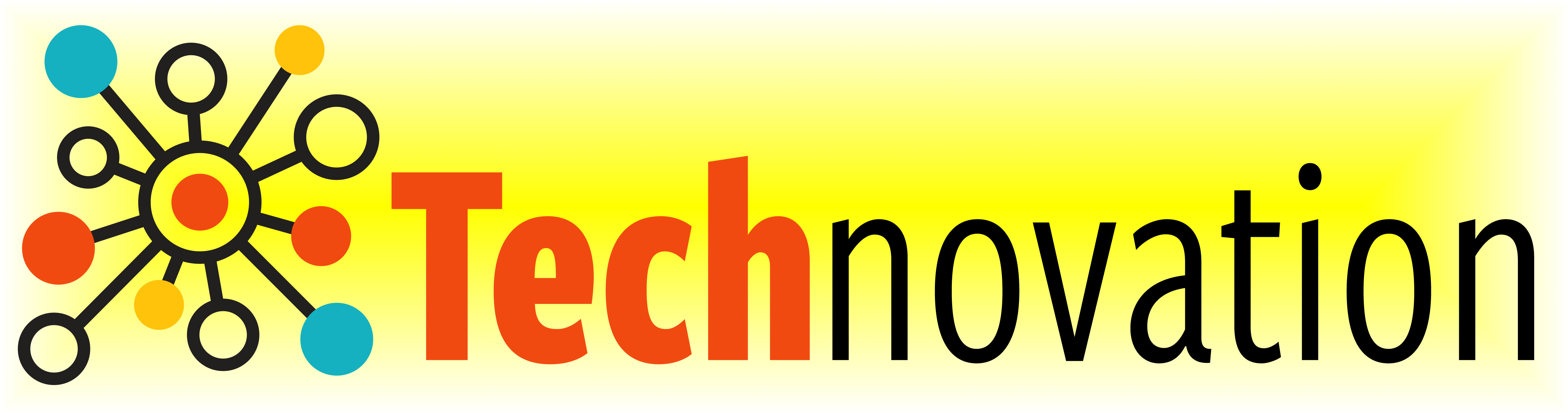# 4.5. Practice Makes Perfect¶

Here’s a reminder of the commands we learned last week:

Command

What does it do?

`forward( distance )`

Move forward a specified distance

`backward( distance )`

Move backward a specified distance

`left(90)`

Turns 90 degrees to the left (you can use any angle, not just 90!)

`right(90)`

Turns 90 degrees to the right

`circle( radius )`

Draws a circle with the specified radius

`goto( x, y )`

Move straight to the position with coordinates (x, y). Note: the center is (0, 0)

`up()`

Stop leaving a trail

`down()`

Start drawing a trail

`color( c )`

Set the color to c (https://trinket.io/docs/colors)

`for i in range( n ):`

Repeat n times

And here are the new commands we’ll be learning this week!

Command

What does it do?

`begin_fill()`

Starts filling in drawn shapes

`end_fill()`

Stops filling in drawn shapes

`speed(number 0-10)`

Determines how quickly the turtle will move through commands

`def function_name():`

Creates and defines a new function

`function_name()`

Calls a function

First, let’s do a quick review of loops by drawing a square using a loop. Then, we can use that code to check out what these new commands do!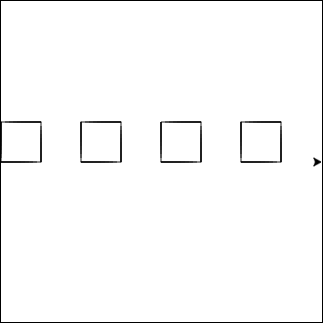Let’s combine what we know about loops with the square function we wrote in the last exercise to draw a row of squares.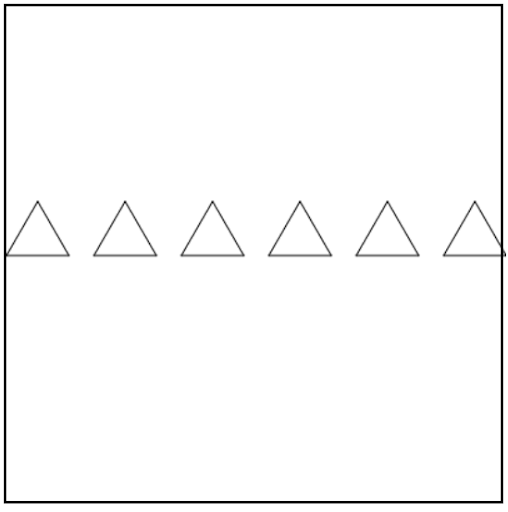Try modifying your code from the previous exercise to draw a row of triangles instead. Feel free to add color or fill in the triangles!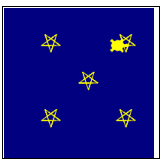Let’s take what we know about loops and functions to draw a starry night sky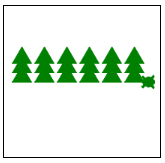How can we use what we know to draw a forest of pine trees?
• How might you draw a forest? Draw a bunch of pine trees!

• How might you draw a pine tree? Draw a stack of triangles!

• How might you draw a stack of triangles? Draw individual triangles offset in a loop!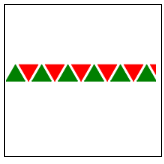Use the starter code below to draw a row of triangles!